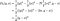4. mirror descent and relative smoothness

Convex non-smooth and the convergence of mirror descent with relative smoothness

back to 《A Deeper Learner》main page

Relative smoothness

In the last lecture, we find smoothness required for convergence guarantee. How to generalize smoothness? Ans: Bregman divergence.

Bregman divergence

Notice that Bregman divergence is not symmetric in terms of x and y, but can be considered an asymmetric “x to y” connotation.Bregman divergence returns to the (second term) of the L-smooth definition.

We can find the second term in the L-smooth requirement as a special case of the Bregman divergence, where h is the half L-scaled-squared Euclidean norm. Thus, by replacing the second term in the L-smooth definition and gradient descent algorithm, we find the relative smoothness and a new type of “mirror” descent algorithm.

Mirror descent convergence proof

The proof follows that of gradient descent and L-smoothness. However, with Bregman divergence, simple concepts (e.g. Pythagorean theorem) now require detailed proofs.

Three-point Equality

no time to write will finish latter

More from Jimmy Yeh

no time to write will finish latter